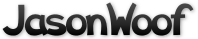Got questions, comments, patches, etc.? Contact Jason Woofenden
diff --git a/draw.h b/draw.h
index 955067d..17d2c89 100644 (file)
--- a/draw.h
+++ b/draw.h
@@ -1,14 +1,5 @@

-/* X11 types - begin */
-struct _XDraw {
-       unsigned int w, h;
-       Display *dpy;
-       Drawable drawable;
-       GC gc;
-};
-typedef struct _XDraw Draw;
-
struct _XCol {
unsigned long rgb;
};
@@ -17,60 +8,57 @@ typedef struct _XCol Col;
struct _XFont {
int ascent;
int descent;
-       unsigned int h, w;
+       unsigned int h;
XFontSet set;
XFontStruct *xfont;
};
typedef struct _XFont Fnt;
-/* X11 types - end */

-typedef struct {
-       Draw *draw;
+typedef struct _XDraw Draw;
+struct _XDraw {
+       unsigned int w, h;
+       Display *dpy;
+       int screen;
+       Window win;
+       Drawable drawable;
+       GC gc;
Col *fg;
Col *bg;
Fnt *font;
-       Bool fill;
-} DDC;
+};

typedef struct {
unsigned int w;
unsigned int h;
-       int x;
-       int y;
int xOff;
int yOff;
} TextExtents;

/* Drawable abstraction */
-Draw *draw_create(Display *dpy, Window win, unsigned int w, unsigned int h);
+Draw *draw_create(Display *dpy, int screen, Window win, unsigned int w, unsigned int h);
void draw_resize(Draw *draw, unsigned int w, unsigned int h);
void draw_free(Draw *draw);

-/* Drawing context abstraction */
-DDC *dc_create(Draw *draw);
-void dc_free(DDC *dc);
-
/* Fnt abstraction */
-Fnt *font_create(const char *fontname);
-void font_free(Fnt *font);
+Fnt *draw_font_create(Draw *draw, const char *fontname);
+void draw_font_free(Draw *draw, Fnt *font);

/* Colour abstraction */
-Col *col_create(const char *colname);
-void col_free(Col *col);
+Col *draw_col_create(Draw *draw, const char *colname);
+void draw_col_free(Draw *draw, Col *col);

/* Drawing context manipulation */
-void dc_setfont(DDC *dc, Fnt *font);
-void dc_setfg(DDC *dc, Col col);
-void dc_setbg(DDC *dc, Col col);
-void dc_setfill(DDC *dc, Bool fill);
+void draw_setfont(Draw *draw, Fnt *font);
+void draw_setfg(Draw *draw, Col *col);
+void draw_setbg(Draw *draw, Col *col);

/* Drawing functions */
-void dc_drawrect(DDC *dc, int x, int y, unsigned int w, unsigned int h);
-void dc_drawtext(DDC *dc, int x, int y, const char *text);
+void draw_rect(Draw *draw, int x, int y, unsigned int w, unsigned int h, Bool filled, Bool empty, Bool invert);
+void draw_text(Draw *draw, int x, int y, unsigned int w, unsigned int h, const char *text, Bool invert);

/* Map functions */
-void dc_map(DDC *dc, int x, int y, unsigned int w, unsigned int h);
+void draw_map(Draw *draw, int x, int y, unsigned int w, unsigned int h);

/* Text functions */
-void dc_getextents(DDC *dc, const char *text, TextExtents *extents);
+void draw_getextents(Draw *draw, const char *text, unsigned int len, TextExtents *extents);